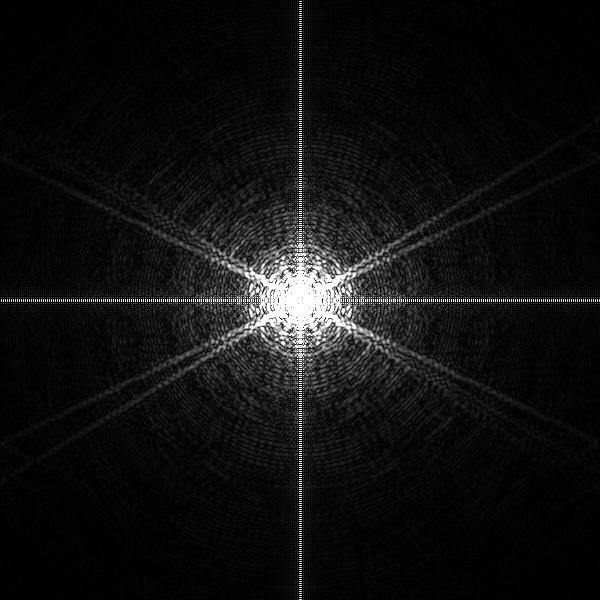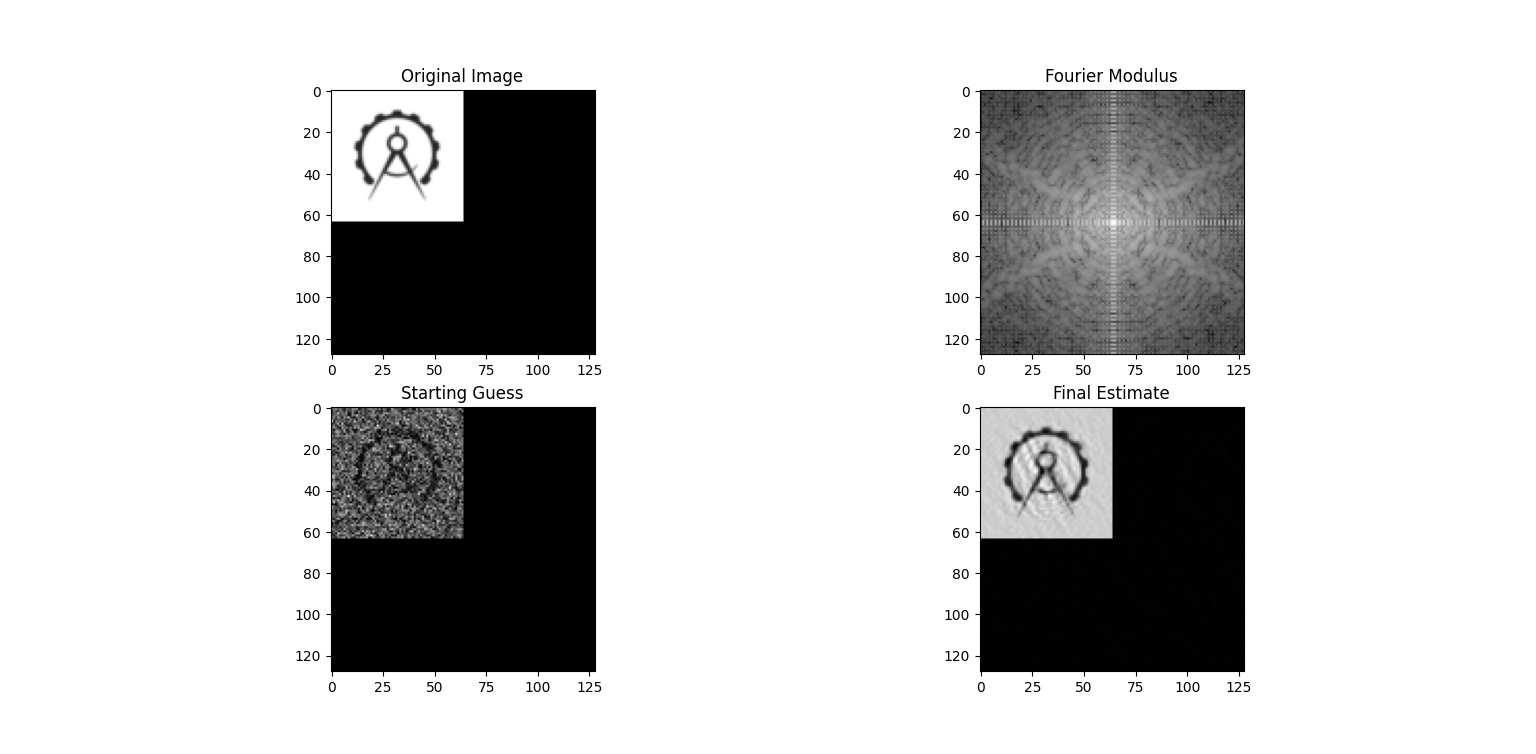## Phase Retrieval

Published 2022/07/12 by Jordan Hay

When light is bent by an object it produces a pattern that can be determined by the discrete Fourier transform of the scattering density of the object (in the direction the light approached from)1. Simply put, how bright an object appears can be used to predict how light will be bent around the object — diffracted by the object. The ‘brightness’ of the diffraction pattern (Figure 1) that we can observe behind the object is determined wholly by the Fourier modulus (the magnitude of the Fourier transform of the object). Diffraction also impacts the phase of the resultant wave. However, measuring the phase of the diffracted wave is a far trickier process than measuring the magnitude.Figure 1 - The Fourier modulus (and thus diffraction pattern) of my logo.

Since we can’t easily measure phase but can magnitude — and crucially phase is needed in order to determine the original image! — methods have been developed to estimate the likely original image iteratively. Central to these algorithms is satisfaction of two opposing constriants 2. Often it is easy to project a guess to satisfy either constraint but to satisfy both (in a timely manner) must be achieved iteratively.

To retrieve phase information we need two key pieces of information. These will form our constraints and we will develop projections to map a guess to match the relevant constraint. The first is the Fourier modulus, a valid estimate of the original image should have a modulus that matches the measured modulus. Second is the support of the object, in order to have constraints out number the free variables (which is a requirement for producing a unique image1) we define areas in which the image cannot exist. For an $N$-pixel image the Fourier modulus has approximately $N/2$ free variables, so the support of the object must not exceed more than half of the image area1.

Now from our two constraints we need form two projections. Projections are maps that apply a transformation to an estimate image that cause it to fulfill the relevant constraint with minimal change to the image. For the modulus constraint we shall call its projection $P_F(x)$ and the support constraint’s projection $P_S(x)$, where $x$ is the estimate image.

We define $P_F$ to perform the following transformation to $x$

1. Produce the fourier transform of $x$, by convention this is $X$
2. Calculate the modulus $M$ of $X$ (the absolute value of each element of $X$)
3. Divide $X$ by $M$ to normalise the values of $X$, then scale $X$ by the measured modulus
4. Inverse the fourier transform of $X$ to produce the new $x$

In Python3

def fourier_projection(image: np.ndarray, target_modulus: np.ndarray) -> np.ndarray:
'''
Performs a minimal modification of the passed image to match the expected Fourier modulus.

Parameters
----------
image: np.ndarray
Image to perform the minimal modification on.
target_modulus: np.ndarray
The expected fourier modulus. These are the magnitudes that the
pixels are scaled to match.
Returns
-------
np.ndarray
The image with minimal modification,
passing it to fourier_modulus should match the target modulus.
'''
fimage = fftn(image)
fimage_modulus = np.abs(fimage)
fimage = (fimage/fimage_modulus) * target_modulus
return ifftn(fimage)

$P_S$ can be defined in far simpler terms. $P_S$ simply takes the image and zeros-out any areas that are not apart of the support of the image. In Python3 this is easily achieved by multiplying by an array where one indicates a supported area, and zero not

def support_projection(image: np.ndarray, support: np.ndarray) -> np.ndarray:
'''
Restricts the image to the domain of its support.
Parameters
----------
image: np.ndarray
The image to constrict the support of.
support: np.ndarray
Boolean array describing areas of support.
Returns
-------
np.ndarray
The image with only supported areas.
'''
return image * support

With two minimal modification projections defined we can begin constructing an iterative method that produces estimates of the image that produced a certain diffraction pattern. Intuitively we can see that alternating the projections should approach a solution, however, this error reduction method often falls into false minimums where it alternates between the same two points rather than approaching a valid solution. Many alternative algorithms have been proposed that attempt avoid these false minima. One such example is ‘the difference map’ and this shall be the example we focus on.

The difference map $D(x)$ is defined

\begin{align*} D(x) &= x + \beta\left[P_F\circ f_S(x) - P_S\circ f_F(x)\right]\\ f_F(x) &= (1 + \gamma_F)P_F(x) - \gamma_F x\\ f_S(x) &= (1 + \gamma_S)P_S(x) - \gamma_S x \end{align*}

Where $\beta$ is a constant that interpolates between the two projections and $\circ$ indicates function composition. $\gamma_F$ and $\gamma_S$ are further constants typically set to $\gamma_F = 1/\beta, \gamma_S = -1/\beta$.

Implementing this in Python3

def difference_map(image: np.ndarray, modulus: np.ndarray, support: np.ndarray) -> np.ndarray:
'''
Executes the difference map described in  and  upon the image once.
Parameters
----------
image: np.ndarray
The image to iterate upon.
modulus: np.ndarray
The fourier modulus the image should be coerced to match.
support: np.ndarray
The support of the image.
Returns
-------
np.ndarray
The image transformed with one iteration of the difference map.
'''
f_F = (1 + Y_F)*fourier_projection(image, modulus) - Y_F*image
f_S = (1 + Y_S)*support_projection(image, support) - Y_S*image
return image + B*(support_projection(f_F, support) - fourier_projection(f_S, modulus))

B/$\beta$, Y_F/$\gamma_F$, Y_S/$\gamma_S$ are globals and defined as in .

With this done it is sufficient to generate a padded guess image and iterate the difference map upon it (Figure 2).Figure 2 - 500 iteration phase retrieval on the Fourier modulus of my logo with a very noisy guess.

Being able to estimate the original image with only the diffraction magnitude is super handy for imaging really small things. This is done by firing a laser of appropriate wavelength through a sample — typically a protein or crystal — and measuring the diffraction pattern.

### Acknowledgements

I am indebted to Joe Chen for introducing me to and teaching me about these topics. Without his guidance on the subject I doubt I would have ever learnt anything about it. Thank you Joe for teaching me something new :)

### Footnotes

1. V. Elser, “The Mermin Fixed Point,” Foundations of Physics, vol. 33, (11), pp. 1691, 2003.  2 3

2. V. Elser, I. Rankenburg and P. Thibault, “Searching with Iterated Maps,” Proceedings of the National Academy of Sciences - PNAS, vol. 104, (2), pp. 418-423, 2007.